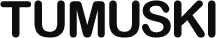Home Page
Pages: 1 2 3 4 5 6

# Clumpy Usage Guide

## Clumpy Has Moved

It’s here now: https://thomasperi.github.io/clumpyjs/control

## Break and Continue

Suppose we wanted to skip all the numbers that are divisible by ten, or if the number meets some special condition, exit the loop.

``````var i;

// Real Loop:
for (i = 0; i < 100000; i++) {
if (i % 10 === 0) {
continue;
}
if (isSpecial(i)) {
break;
}
// statements
}

// Clumpy Equivalent:
clumpy.for_loop(
function () { i = 0; },
function () { return i < 100000; },
function () { i++; },
function () {
if (i % 10 === 0) {
clumpy.continue_loop();
return;
}
if (isSpecial(i)) {
clumpy.break_loop();
return;
}
// statements
}
);``````

This is where there’s the greatest likelihood of error. Notice the `return;` after the `clumpy.continue_loop();` statement. This is because if we don’t explicitly leave the function, JavaScript will continue to execute the rest of it.

I’ve found that a good pattern to follow is to put the `return` before the method invocation in the same statement (returning the result) instead of after it in a separate statement:

``````var i;

return clumpy.
for_loop(
function () { i = 0; },
function () { return i < 100000; },
function () { i++; },
function () {
if (i % 10 === 0) {
return clumpy.
continue_loop();
}
if (isSpecial(i)) {
return clumpy.
break_loop();
}
// statements
}
).
once(function () {
// etc.
});``````

Next Page: Labels

© Thomas Peri